# 17. Machine design¶

This week assignment - actuate and automate your machine - document the group project and your individual contribution

Group Page

## My Contribution¶

• Turntable design & fabrication
• Demo video making

## Make a turntable¶

For this week we decided to create a robot hand and I was in charge of creating the basement part which is a turntable.

A turntble is for moving robot arm horizontally which is moved stepping motor & timing belt. This is controlled by Arduino.

On week 15 I designed basement to cut the part by a laser cutter and this week we got the parts so we assembled to make it movable.

First, I put 5 gears together since we need the height to adjust the heights 2 stepping motor. so we decided to assemble it with glue. so before make it production we tested several types of glues. one is 10 seconds and the other one is in 2 minutes. so we use a small part small part of acryl part with 10-second glue and it seems like too fast to getting together because we need to adjust the gears accurately so then I tested 2 minutes-glue.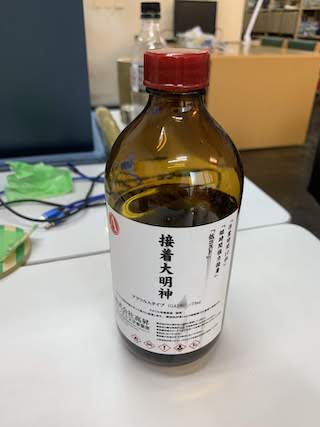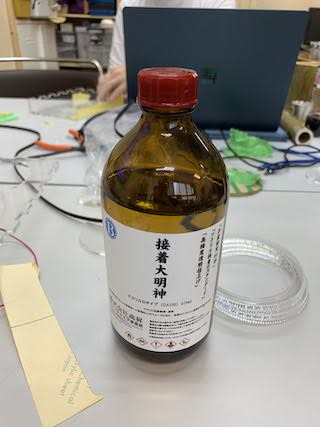how many school is also checking because after touching to part it moves easily so I have to be cautious I decided to use 2 minutes glue for gears. So finally we move to actual production of gears and I put it on gears and assemble it.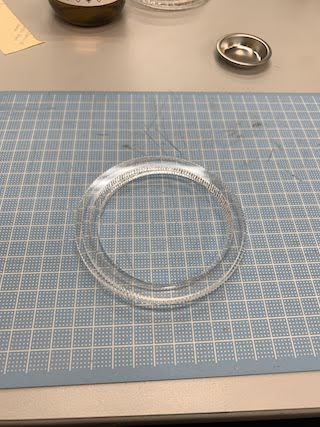the tricky part was that years on not 100% straight so some gears tilt a little bit. so I carefully assembled 5 gears and also basement and And the spindle part.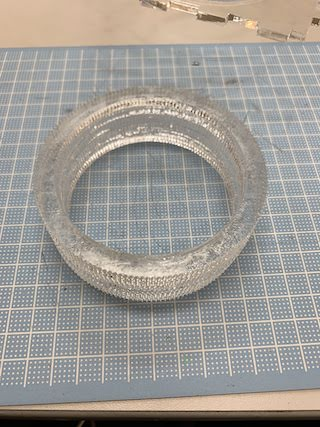after implement these part I tested if it turns easily and actually didn’t because I put it’s a little bit too far from stepping motor because I intended because timing belt to stepping motor side as I said it was too far and the some parts of it hit the hole so I need to use sandpaper to create this.

after. It turns free functionally so so I moved to setting timing belt with the gears and the stepping motors.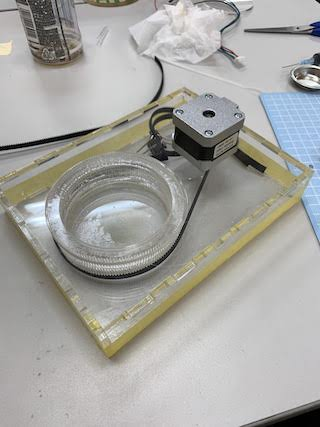firstly I cut the timing belt for this machine and Tie The End by plastic belt. but plastic belt was gradually loosen cuz of the gear movement so you know the two getting together tightly I made a hole on timing belt and put it together with screw.

it worked out so I got now turntable movement function. and then I put the top part of the turntable with glue again. after that I combined arm part with the turntable by 4 screw. finally we made a robot arm.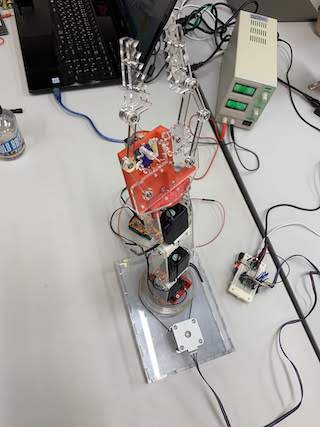## Demo video¶

Made a demo video with iMovie and ffmpeg.

First, put a movie into iMovie.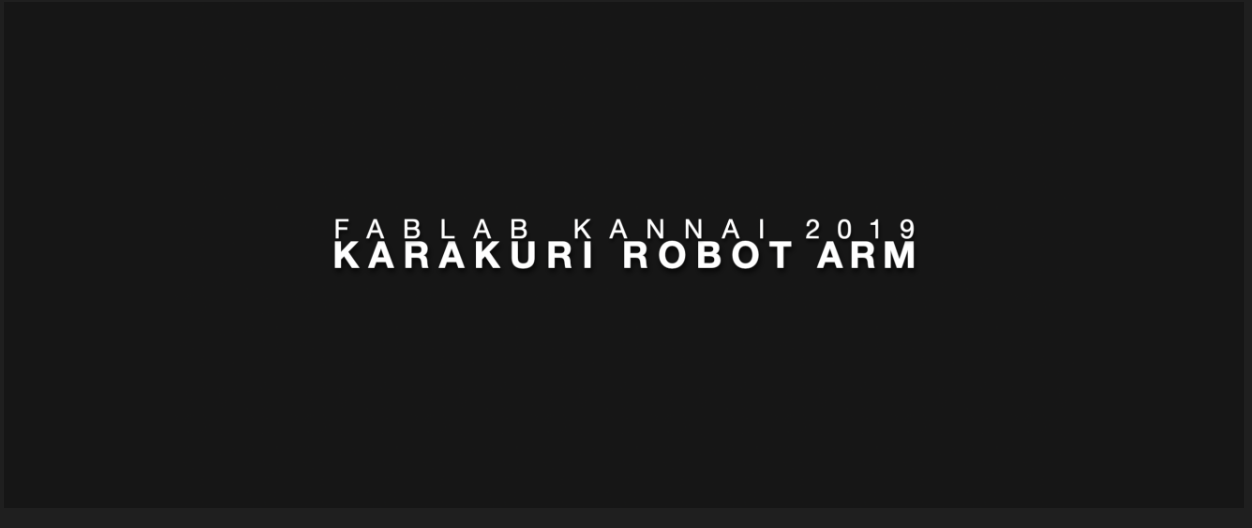Then, added title effect and edit text.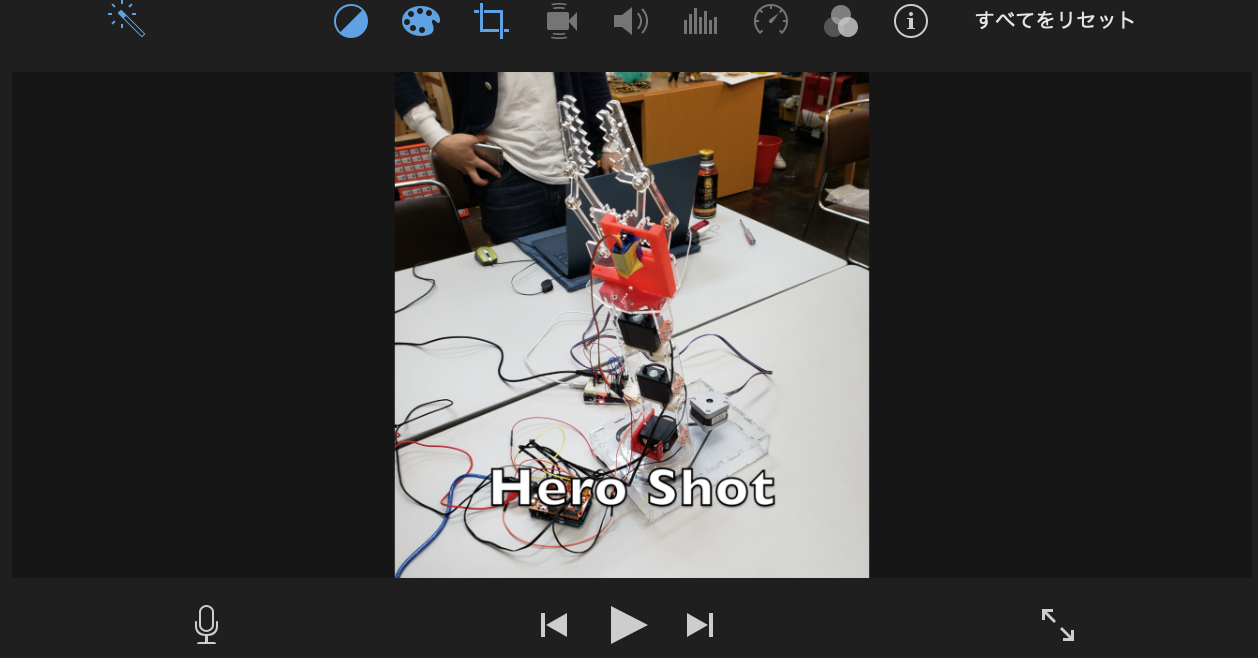After that, added endroll with the credit with sound named news from library.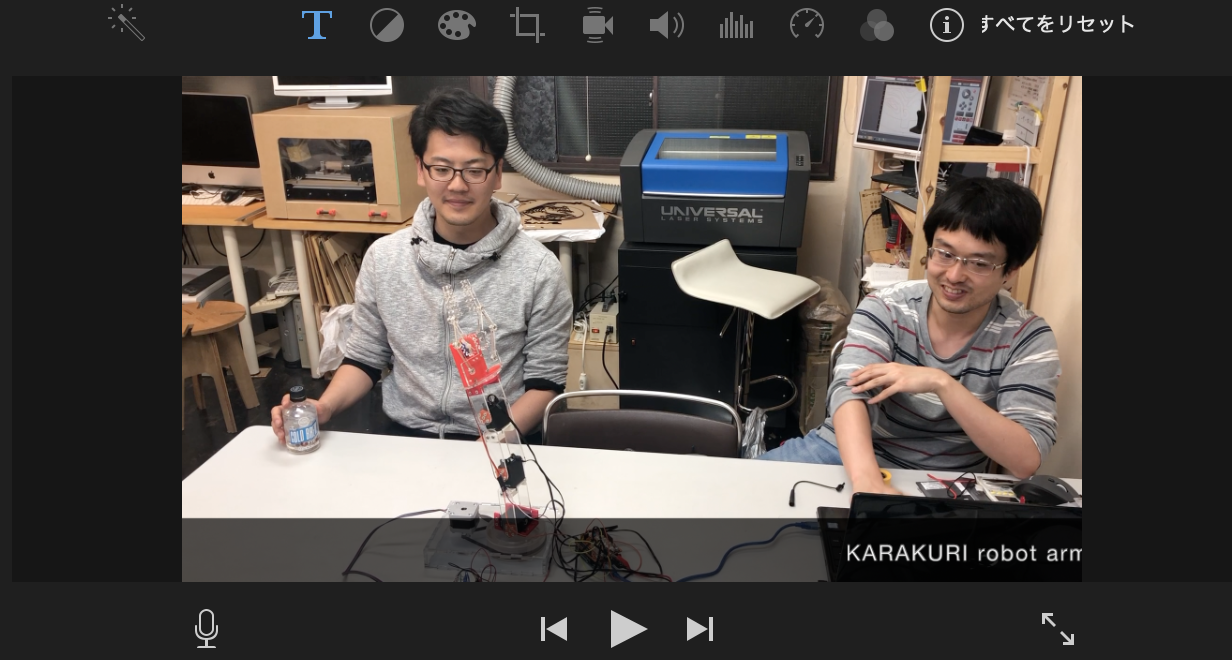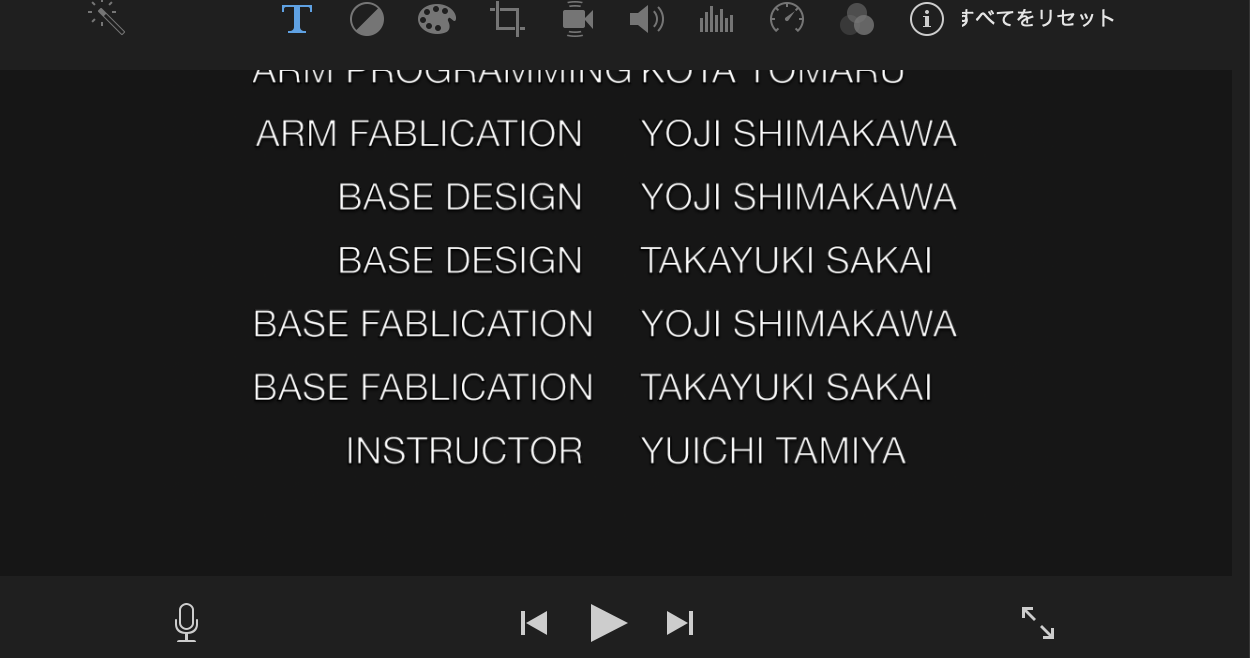This is the finish of editting. So I selected export to make mpeg 4.

## ffmpeg¶

In order to compress the movie I made on iMovie, I use ffmpeg.

First intstall ffmpeg on Terminal with Homebrew.

```brew install ffmpeg
```

This time, format was fixed to max 1min, mp4, 1920:1080, under 10MB. So I run a following code on Terminal

```ffmpeg \
-i fabmovie.mp4 \
-vf "scale=1920:1080" \
-c:v mpeg4 \
-q:v 16 \
outputMovie.mp4
```

-i means original file name -vf means size -c means file format -q means compress level

but this didn’t work because file size was still 96.4MB.

so then I tried this.

```ffmpeg -i fabmovie3.mp4 -vf "scale=1920:1080,pad=1920:1080:0:0:black" -c:v mpeg4 -q:v 256 outputMovie.mp4
```

But still 14MB. So I tried to reduce the size of original file. I choose lowest quality when I exported on iMovie, then hit this.

```ffmpeg -i fabmovie2.mp4 -vf "scale=1920:1080,pad=1920:1080:0:0:black" -crf 32 outputMovie.mp4 -vcodec h264
ffmpeg version 4.1.3 Copyright (c) 2000-2019 the FFmpeg developers
built with Apple LLVM version 10.0.1 (clang-1001.0.46.4)
configuration: --prefix=/usr/local/Cellar/ffmpeg/4.1.3_1 --enable-shared --enable-pthreads --enable-version3 --enable-hardcoded-tables --enable-avresample --cc=clang --host-cflags='-I/Library/Java/JavaVirtualMachines/adoptopenjdk-11.0.2.jdk/Contents/Home/include -I/Library/Java/JavaVirtualMachines/adoptopenjdk-11.0.2.jdk/Contents/Home/include/darwin' --host-ldflags= --enable-ffplay --enable-gnutls --enable-gpl --enable-libaom --enable-libbluray --enable-libmp3lame --enable-libopus --enable-librubberband --enable-libsnappy --enable-libtesseract --enable-libtheora --enable-libvorbis --enable-libvpx --enable-libx264 --enable-libx265 --enable-libxvid --enable-lzma --enable-libfontconfig --enable-libfreetype --enable-frei0r --enable-libass --enable-libopencore-amrnb --enable-libopencore-amrwb --enable-libopenjpeg --enable-librtmp --enable-libspeex --enable-videotoolbox --disable-libjack --disable-indev=jack --enable-libaom --enable-libsoxr
libavutil      56. 22.100 / 56. 22.100
libavcodec     58. 35.100 / 58. 35.100
libavformat    58. 20.100 / 58. 20.100
libavdevice    58.  5.100 / 58.  5.100
libavfilter     7. 40.101 /  7. 40.101
libavresample   4.  0.  0 /  4.  0.  0
libswscale      5.  3.100 /  5.  3.100
libswresample   3.  3.100 /  3.  3.100
libpostproc    55.  3.100 / 55.  3.100
Trailing options were found on the commandline.
Input #0, mov,mp4,m4a,3gp,3g2,mj2, from 'fabmovie2.mp4':
major_brand     : mp42
minor_version   : 1
compatible_brands: mp41mp42isom
creation_time   : 2019-05-22T09:39:09.000000Z
Duration: 00:01:00.86, start: 0.000000, bitrate: 6629 kb/s
Stream #0:0(jpn): Audio: aac (LC) (mp4a / 0x6134706D), 48000 Hz, stereo, fltp, 98 kb/s (default)
creation_time   : 2019-05-22T09:39:09.000000Z
handler_name    : Core Media Audio
Stream #0:1(und): Video: h264 (High) (avc1 / 0x31637661), yuv420p(tv, bt709, progressive), 1920x1080 [SAR 1:1 DAR 16:9], 6522 kb/s, 29.97 fps, 29.97 tbr, 30k tbn, 60k tbc (default)
creation_time   : 2019-05-22T09:39:09.000000Z
handler_name    : Core Media Video
File 'outputMovie.mp4' already exists. Overwrite ? [y/N] y
Stream mapping:
Stream #0:1 -> #0:0 (h264 (native) -> h264 (libx264))
Stream #0:0 -> #0:1 (aac (native) -> aac (native))
Press [q] to stop, [?] for help
[libx264 @ 0x7fa7ab823a00] using SAR=1/1
[libx264 @ 0x7fa7ab823a00] using cpu capabilities: MMX2 SSE2Fast SSSE3 SSE4.2 AVX FMA3 BMI2 AVX2
[libx264 @ 0x7fa7ab823a00] profile High, level 4.0
[libx264 @ 0x7fa7ab823a00] 264 - core 155 r2917 0a84d98 - H.264/MPEG-4 AVC codec - Copyleft 2003-2018 - http://www.videolan.org/x264.html - options: cabac=1 ref=3 deblock=1:0:0 analyse=0x3:0x113 me=hex subme=7 psy=1 psy_rd=1.00:0.00 mixed_ref=1 me_range=16 chroma_me=1 trellis=1 8x8dct=1 cqm=0 deadzone=21,11 fast_pskip=1 chroma_qp_offset=-2 threads=6 lookahead_threads=1 sliced_threads=0 nr=0 decimate=1 interlaced=0 bluray_compat=0 constrained_intra=0 bframes=3 b_pyramid=2 b_adapt=1 b_bias=0 direct=1 weightb=1 open_gop=0 weightp=2 keyint=250 keyint_min=25 scenecut=40 intra_refresh=0 rc_lookahead=40 rc=crf mbtree=1 crf=32.0 qcomp=0.60 qpmin=0 qpmax=69 qpstep=4 ip_ratio=1.40 aq=1:1.00
Output #0, mp4, to 'outputMovie.mp4':
major_brand     : mp42
minor_version   : 1
compatible_brands: mp41mp42isom
encoder         : Lavf58.20.100
Stream #0:0(und): Video: h264 (libx264) (avc1 / 0x31637661), yuv420p, 1920x1080 [SAR 1:1 DAR 16:9], q=-1--1, 29.97 fps, 30k tbn, 29.97 tbc (default)
creation_time   : 2019-05-22T09:39:09.000000Z
handler_name    : Core Media Video
encoder         : Lavc58.35.100 libx264
Side data:
cpb: bitrate max/min/avg: 0/0/0 buffer size: 0 vbv_delay: -1
Stream #0:1(jpn): Audio: aac (LC) (mp4a / 0x6134706D), 48000 Hz, stereo, fltp, 128 kb/s (default)
creation_time   : 2019-05-22T09:39:09.000000Z
handler_name    : Core Media Audio
encoder         : Lavc58.35.100 aac
frame=   41 fps=0.0 q=0.0 size=       0kB time=00:00:02.45 bitrate=   0.2kbits/sframe=   50 fps= 50 q=0.0 size=       0kB time=00:00:02.75 bitrate=   0.1kbits/sframe=   60 fps= 38 q=38.0 size=       0kB time=00:00:03.09 bitrate=   0.1kbits/frame=   83 fps= 39 q=38.0 size=       0kB time=00:00:03.86 bitrate=   0.1kbits/frame=  106 fps= 41 q=38.0 size=       0kB time=00:00:04.62 bitrate=   0.1kbits/frame=  132 fps= 42 q=38.0 size=       0kB time=00:00:05.50 bitrate=   0.1kbits/frame=  163 fps= 45 q=38.0 size=       0kB time=00:00:06.52 bitrate=   0.1kbits/frame=  186 fps= 44 q=38.0 size=       0kB time=00:00:07.29 bitrate=   0.1kbits/frame=  214 fps= 45 q=38.0 size=       0kB time=00:00:08.23 bitrate=   0.0kbits/frame=  236 fps= 45 q=38.0 size=       0kB time=00:00:08.96 bitrate=   0.0kbits/frame=  258 fps= 45 q=38.0 size=       0kB time=00:00:09.70 bitrate=   0.0kbits/frame=  287 fps= 45 q=38.0 size=       0kB time=00:00:10.66 bitrate=   0.0kbits/frame=  324 fps= 47 q=38.0 size=       0kB time=00:00:11.90 bitrate=   0.0kbits/frame=  355 fps= 48 q=38.0 size=       0kB time=00:00:12.92 bitrate=   0.0kbits/frame=  374 fps= 48 q=38.0 size=       0kB time=00:00:13.56 bitrate=   0.0kbits/frame=  387 fps= 46 q=38.0 size=       0kB time=00:00:13.99 bitrate=   0.0kbits/frame=  404 fps= 45 q=38.0 size=       0kB time=00:00:14.57 bitrate=   0.0kbits/frame=  411 fps= 43 q=38.0 size=     256kB time=00:00:14.80 bitrate= 141.7kbits/frame=  423 fps= 42 q=38.0 size=     256kB time=00:00:15.21 bitrate= 137.9kbits/frame=  435 fps= 40 q=38.0 size=     256kB time=00:00:15.59 bitrate= 134.5kbits/frame=  447 fps= 39 q=38.0 size=     256kB time=00:00:16.00 bitrate= 131.1kbits/frame=  459 fps= 38 q=38.0 size=     256kB time=00:00:16.40 bitrate= 127.9kbits/frame=  471 fps= 37 q=38.0 size=     256kB time=00:00:16.81 bitrate= 124.8kbits/frame=  483 fps= 36 q=38.0 size=     512kB time=00:00:17.21 bitrate= 243.7kbits/frame=  495 fps= 35 q=38.0 size=     512kB time=00:00:17.60 bitrate= 238.3kbits/frame=  505 fps= 35 q=38.0 size=     512kB time=00:00:17.94 bitrate= 233.8kbits/frame=  515 fps= 34 q=38.0 size=     768kB time=00:00:18.28 bitrate= 344.1kbits/frame=  527 fps= 34 q=38.0 size=     768kB time=00:00:18.66 bitrate= 337.1kbits/frame=  533 fps= 33 q=38.0 size=     768kB time=00:00:18.88 bitrate= 333.3kbits/frame=  546 fps= 32 q=38.0 size=     768kB time=00:00:19.30 bitrate= 325.9kbits/frame=  559 fps= 32 q=38.0 size=    1024kB time=00:00:19.73 bitrate= 425.1kbits/frame=  569 fps= 32 q=38.0 size=    1024kB time=00:00:20.07 bitrate= 417.9kbits/frame=  583 fps= 32 q=38.0 size=    1024kB time=00:00:20.54 bitrate= 408.3kbits/frame=  597 fps= 31 q=38.0 size=    1024kB time=00:00:21.01 bitrate= 399.2kbits/frame=  609 fps= 31 q=38.0 size=    1280kB time=00:00:21.41 bitrate= 489.6kbits/frame=  621 fps= 31 q=38.0 size=    1280kB time=00:00:21.80 bitrate= 481.0kbits/frame=  632 fps= 31 q=38.0 size=    1280kB time=00:00:22.18 bitrate= 472.6kbits/frame=  643 fps= 30 q=38.0 size=    1280kB time=00:00:22.54 bitrate= 465.0kbits/frame=  653 fps= 30 q=38.0 size=    1536kB time=00:00:22.86 bitrate= 550.2kbits/frame=  662 fps= 30 q=38.0 size=    1536kB time=00:00:23.16 bitrate= 543.1kbits/frame=  671 fps= 30 q=38.0 size=    1536kB time=00:00:23.48 bitrate= 535.7kbits/frame=  683 fps= 29 q=38.0 size=    1792kB time=00:00:23.87 bitrate= 615.0kbits/frame=  694 fps= 29 q=38.0 size=    1792kB time=00:00:24.25 bitrate= 605.2kbits/frame=  703 fps= 29 q=38.0 size=    1792kB time=00:00:24.55 bitrate= 597.9kbits/frame=  713 fps= 29 q=38.0 size=    2048kB time=00:00:24.87 bitrate= 674.5kbits/frame=  722 fps= 28 q=38.0 size=    2048kB time=00:00:25.17 bitrate= 666.5kbits/frame=  733 fps= 28 q=38.0 size=    2048kB time=00:00:25.55 bitrate= 656.5kbits/frame=  743 fps= 28 q=38.0 size=    2304kB time=00:00:25.87 bitrate= 729.4kbits/frame=  753 fps= 28 q=38.0 size=    2304kB time=00:00:26.21 bitrate= 719.9kbits/frame=  765 fps= 28 q=38.0 size=    2304kB time=00:00:26.62 bitrate= 708.9kbits/frame=  775 fps= 27 q=38.0 size=    2560kB time=00:00:26.94 bitrate= 778.4kbits/frame=  786 fps= 27 q=38.0 size=    2560kB time=00:00:27.30 bitrate= 768.0kbits/frame=  796 fps= 27 q=38.0 size=    2560kB time=00:00:27.62 bitrate= 759.1kbits/frame=  806 fps= 27 q=38.0 size=    2560kB time=00:00:27.98 bitrate= 749.3kbits/frame=  817 fps= 27 q=38.0 size=    2816kB time=00:00:28.35 bitrate= 813.7kbits/frame=  831 fps= 27 q=38.0 size=    2816kB time=00:00:28.82 bitrate= 800.4kbits/frame=  839 fps= 27 q=38.0 size=    2816kB time=00:00:29.07 bitrate= 793.4kbits/frame=  848 fps= 26 q=38.0 size=    2816kB time=00:00:29.37 bitrate= 785.3kbits/frame=  861 fps= 26 q=38.0 size=    3072kB time=00:00:29.82 bitrate= 843.8kbits/frame=  874 fps= 26 q=38.0 size=    3072kB time=00:00:30.25 bitrate= 831.9kbits/frame=  888 fps= 26 q=38.0 size=    3072kB time=00:00:30.72 bitrate= 819.2kbits/frame=  897 fps= 26 q=38.0 size=    3072kB time=00:00:31.01 bitrate= 811.3kbits/frame=  911 fps= 26 q=38.0 size=    3328kB time=00:00:31.48 bitrate= 865.8kbits/frame=  925 fps= 26 q=38.0 size=    3328kB time=00:00:31.95 bitrate= 853.1kbits/frame=  935 fps= 26 q=38.0 size=    3328kB time=00:00:32.27 bitrate= 844.7kbits/frame=  943 fps= 26 q=38.0 size=    3584kB time=00:00:32.55 bitrate= 901.9kbits/frame=  954 fps= 26 q=38.0 size=    3584kB time=00:00:32.91 bitrate= 891.9kbits/frame=  964 fps= 26 q=38.0 size=    3584kB time=00:00:33.25 bitrate= 882.8kbits/frame=  973 fps= 26 q=38.0 size=    3840kB time=00:00:33.55 bitrate= 937.4kbits/frame=  981 fps= 25 q=38.0 size=    3840kB time=00:00:33.81 bitrate= 930.3kbits/frame=  989 fps= 25 q=38.0 size=    3840kB time=00:00:34.09 bitrate= 922.8kbits/frame= 1000 fps= 25 q=38.0 size=    4096kB time=00:00:34.45 bitrate= 973.9kbits/frame= 1010 fps= 25 q=38.0 size=    4096kB time=00:00:34.79 bitrate= 964.4kbits/frame= 1015 fps= 25 q=38.0 size=    4096kB time=00:00:34.96 bitrate= 959.7kbits/frame= 1024 fps= 25 q=38.0 size=    4352kB time=00:00:35.26 bitrate=1011.0kbits/frame= 1033 fps= 25 q=38.0 size=    4352kB time=00:00:35.56 bitrate=1002.5kbits/frame= 1043 fps= 25 q=38.0 size=    4352kB time=00:00:35.88 bitrate= 993.6kbits/frame= 1052 fps= 25 q=38.0 size=    4608kB time=00:00:36.18 bitrate=1043.3kbits/frame= 1062 fps= 24 q=38.0 size=    4608kB time=00:00:36.52 bitrate=1033.6kbits/frame= 1072 fps= 24 q=38.0 size=    4608kB time=00:00:36.86 bitrate=1024.0kbits/frame= 1083 fps= 24 q=38.0 size=    4864kB time=00:00:37.22 bitrate=1070.4kbits/frame= 1095 fps= 24 q=38.0 size=    4864kB time=00:00:37.63 bitrate=1058.8kbits/frame= 1108 fps= 24 q=38.0 size=    4864kB time=00:00:38.05 bitrate=1047.0kbits/frame= 1118 fps= 24 q=38.0 size=    4864kB time=00:00:38.40 bitrate=1037.7kbits/frame= 1131 fps= 24 q=38.0 size=    5120kB time=00:00:38.82 bitrate=1080.3kbits/frame= 1143 fps= 24 q=38.0 size=    5120kB time=00:00:39.23 bitrate=1069.1kbits/frame= 1155 fps= 24 q=38.0 size=    5120kB time=00:00:39.63 bitrate=1058.2kbits/frame= 1169 fps= 24 q=38.0 size=    5376kB time=00:00:40.08 bitrate=1098.7kbits/frame= 1182 fps= 24 q=38.0 size=    5376kB time=00:00:40.53 bitrate=1086.5kbits/frame= 1195 fps= 24 q=38.0 size=    5376kB time=00:00:40.96 bitrate=1075.2kbits/frame= 1206 fps= 24 q=38.0 size=    5376kB time=00:00:41.32 bitrate=1065.8kbits/frame= 1218 fps= 24 q=38.0 size=    5376kB time=00:00:41.72 bitrate=1055.4kbits/frame= 1228 fps= 24 q=38.0 size=    5632kB time=00:00:42.06 bitrate=1096.7kbits/frame= 1241 fps= 24 q=38.0 size=    5632kB time=00:00:42.49 bitrate=1085.7kbits/frame= 1253 fps= 24 q=38.0 size=    5632kB time=00:00:42.90 bitrate=1075.4kbits/frame= 1264 fps= 24 q=38.0 size=    5632kB time=00:00:43.26 bitrate=1066.4kbits/frame= 1275 fps= 24 q=38.0 size=    5888kB time=00:00:43.62 bitrate=1105.6kbits/frame= 1285 fps= 24 q=38.0 size=    5888kB time=00:00:43.96 bitrate=1097.0kbits/frame= 1297 fps= 24 q=38.0 size=    5888kB time=00:00:44.37 bitrate=1087.0kbits/frame= 1309 fps= 24 q=38.0 size=    6144kB time=00:00:44.75 bitrate=1124.6kbits/frame= 1323 fps= 24 q=38.0 size=    6144kB time=00:00:45.22 bitrate=1112.9kbits/frame= 1335 fps= 24 q=38.0 size=    6144kB time=00:00:45.63 bitrate=1103.0kbits/frame= 1344 fps= 24 q=38.0 size=    6400kB time=00:00:45.93 bitrate=1141.5kbits/frame= 1357 fps= 24 q=38.0 size=    6400kB time=00:00:46.37 bitrate=1130.5kbits/frame= 1367 fps= 24 q=38.0 size=    6400kB time=00:00:46.69 bitrate=1122.7kbits/frame= 1378 fps= 23 q=38.0 size=    6656kB time=00:00:47.06 bitrate=1158.6kbits/frame= 1388 fps= 23 q=38.0 size=    6656kB time=00:00:47.40 bitrate=1150.3kbits/frame= 1400 fps= 23 q=38.0 size=    6656kB time=00:00:47.80 bitrate=1140.5kbits/frame= 1407 fps= 23 q=38.0 size=    6656kB time=00:00:48.04 bitrate=1135.0kbits/frame= 1417 fps= 23 q=38.0 size=    6912kB time=00:00:48.36 bitrate=1170.8kbits/frame= 1429 fps= 23 q=38.0 size=    6912kB time=00:00:48.76 bitrate=1161.1kbits/frame= 1437 fps= 23 q=38.0 size=    6912kB time=00:00:49.04 bitrate=1154.5kbits/frame= 1447 fps= 23 q=38.0 size=    7168kB time=00:00:49.36 bitrate=1189.5kbits/frame= 1454 fps= 23 q=38.0 size=    7168kB time=00:00:49.60 bitrate=1183.9kbits/frame= 1462 fps= 23 q=38.0 size=    7168kB time=00:00:49.87 bitrate=1177.3kbits/frame= 1471 fps= 23 q=38.0 size=    7424kB time=00:00:50.17 bitrate=1212.1kbits/frame= 1478 fps= 23 q=38.0 size=    7424kB time=00:00:50.41 bitrate=1206.4kbits/frame= 1488 fps= 23 q=38.0 size=    7424kB time=00:00:50.73 bitrate=1198.8kbits/frame= 1497 fps= 23 q=38.0 size=    7680kB time=00:00:51.02 bitrate=1232.9kbits/frame= 1507 fps= 23 q=38.0 size=    7680kB time=00:00:51.37 bitrate=1224.7kbits/frame= 1518 fps= 23 q=38.0 size=    7936kB time=00:00:51.73 bitrate=1256.7kbits/frame= 1528 fps= 23 q=38.0 size=    7936kB time=00:00:52.07 bitrate=1248.4kbits/frame= 1540 fps= 23 q=38.0 size=    7936kB time=00:00:52.48 bitrate=1238.8kbits/frame= 1551 fps= 23 q=38.0 size=    8192kB time=00:00:52.84 bitrate=1270.0kbits/frame= 1563 fps= 23 q=38.0 size=    8192kB time=00:00:53.24 bitrate=1260.3kbits/frame= 1574 fps= 23 q=38.0 size=    8192kB time=00:00:53.61 bitrate=1251.8kbits/frame= 1586 fps= 23 q=38.0 size=    8192kB time=00:00:54.01 bitrate=1242.4kbits/frame= 1600 fps= 23 q=38.0 size=    8448kB time=00:00:54.48 bitrate=1270.2kbits/frame= 1614 fps= 23 q=38.0 size=    8448kB time=00:00:54.93 bitrate=1259.8kbits/frame= 1630 fps= 23 q=38.0 size=    8448kB time=00:00:55.44 bitrate=1248.2kbits/frame= 1647 fps= 23 q=38.0 size=    8448kB time=00:00:56.04 bitrate=1234.9kbits/frame= 1669 fps= 23 q=38.0 size=    8448kB time=00:00:56.76 bitrate=1219.1kbits/frame= 1689 fps= 23 q=38.0 size=    8448kB time=00:00:57.45 bitrate=1204.6kbits/frame= 1709 fps= 23 q=38.0 size=    8704kB time=00:00:58.11 bitrate=1227.0kbits/frame= 1728 fps= 23 q=38.0 size=    8704kB time=00:00:58.75 bitrate=1213.6kbits/frame= 1751 fps= 23 q=38.0 size=    8704kB time=00:00:59.52 bitrate=1198.0kbits/frame= 1776 fps= 24 q=38.0 size=    8704kB time=00:01:00.35 bitrate=1181.5kbits/frame= 1807 fps= 24 q=38.0 size=    8960kB time=00:01:00.82 bitrate=1206.8kbits/frame= 1824 fps= 24 q=-1.0 Lsize=    9091kB time=00:01:00.86 bitrate=1223.6kbits/s speed=0.797x
[libx264 @ 0x7fa7ab823a00] frame I:8     Avg QP:24.42  size: 54294
[libx264 @ 0x7fa7ab823a00] frame P:902   Avg QP:30.29  size:  6683
[libx264 @ 0x7fa7ab823a00] frame B:914   Avg QP:31.35  size:  2177
[libx264 @ 0x7fa7ab823a00] consecutive B-frames: 25.9% 17.4% 13.0% 43.6%
[libx264 @ 0x7fa7ab823a00] mb I  I16..4: 44.2% 41.2% 14.5%
[libx264 @ 0x7fa7ab823a00] mb P  I16..4:  2.3%  4.6%  0.2%  P16..4: 20.9%  2.1%  0.8%  0.0%  0.0%    skip:69.1%
[libx264 @ 0x7fa7ab823a00] mb B  I16..4:  0.2%  0.5%  0.0%  B16..8: 19.0%  0.5%  0.0%  direct: 0.1%  skip:79.6%  L0:44.5% L1:54.8% BI: 0.7%
[libx264 @ 0x7fa7ab823a00] 8x8 transform intra:62.3% inter:89.6%
[libx264 @ 0x7fa7ab823a00] coded y,uvDC,uvAC intra: 15.5% 19.6% 1.5% inter: 2.1% 1.3% 0.0%
[libx264 @ 0x7fa7ab823a00] i16 v,h,dc,p: 36% 40% 12% 13%
[libx264 @ 0x7fa7ab823a00] i8 v,h,dc,ddl,ddr,vr,hd,vl,hu: 16% 14% 55%  2%  3%  3%  4%  2%  2%
[libx264 @ 0x7fa7ab823a00] i4 v,h,dc,ddl,ddr,vr,hd,vl,hu: 23% 24% 21%  4%  7%  6%  8%  4%  3%
[libx264 @ 0x7fa7ab823a00] i8c dc,h,v,p: 75% 15% 10%  1%
[libx264 @ 0x7fa7ab823a00] Weighted P-Frames: Y:4.7% UV:0.7%
[libx264 @ 0x7fa7ab823a00] ref P L0: 66.2% 18.0% 12.0%  3.8%  0.1%
[libx264 @ 0x7fa7ab823a00] ref B L0: 87.3% 10.8%  1.9%
[libx264 @ 0x7fa7ab823a00] ref B L1: 97.5%  2.5%
[libx264 @ 0x7fa7ab823a00] kb/s:1111.03
[aac @ 0x7fa7ab825200] Qavg: 13191.075
```

Finally I can get a file has only 9.3MB.